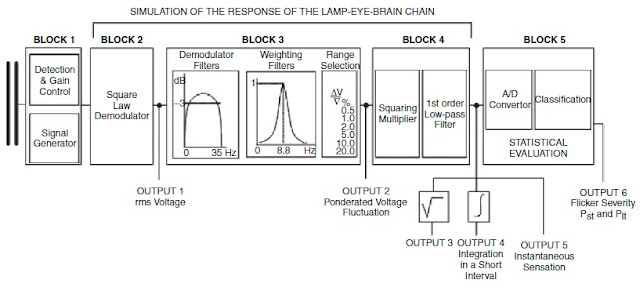## Saturday, September 17, 2011

The UIE/IEC Flickermeter is the standard for flicker measurement. Its main function is to provide assessment of the flicker perception caused by voltage fluctuations. Therefore, the flickermeter should be designed to have the capability of transforming the input voltage fluctuations into an output parameter proportionally related to flicker perception. This is done by simulating the process of physiological visual perception, which is the lamp–eye–brain chain.

The UIE/IEC flickermeter consists of five blocks, which are defined in IEC 61000-4-15 (Testing and Measurement Techniques – Flickermeter – Functional and Design Specifications). It was originally designed using a 60 W, 230 V, incandescent lamp. Recently though, an extended measurement for 120 V was added to cater such electrical systems just like in the United States (see IEEE 1453-2004).

The following sections described each block of the UIE/IEC flickermeter with respect to the signal processing functions provided by the block and to the relationship corresponding to physiological phenomena.

Block 1 is the input voltage adapter, which scales the input half-cycle RMS voltage to an internal reference value. The primary function of this block is to allow flicker measurements be expressed as a percent ratio and become independent of the input voltage level.

An automatic gain control circuit with a 10% to 90% step-response characteristic of one minute gives such functionality. It imitates a well-known characteristic of human perception wherein moderate-level, constant stimuli to the senses slowly become unnoticeable.

2. Square Law or Quadratic Demodulator

Block 2 of the IEC flickermeter is a quadratic demodulator, which squares the scaled input in order to separate the voltage fluctuation (modulating signal) from the main voltage signal. This process simulates the behavior of an incandescent lamp. In other words, the objective of this block is to recapture the modulating signals while at the same time suppress the mains frequency carrier signal.UIE/IEC Flickermeter Block Diagram
3. Demodulator and Weighting Filters

Block 3 of the IEC flickermeter consists of three cascaded filters that serve to filter out components that have frequencies higher than that of the supply voltage and the DC component, produced from the square law demodulator. It also functions to weight the input signal according to the incandescent lamp eye-brain response. These filters are:

a.    Demodulator Filters
• First-order high-pass (cutoff frequency = 0.05 Hz)
• Sixth-order low-pass Butterworth (cutoff frequency = 35 Hz)
b. Weighting Filter
• Band-pass filter (models the frequency-selective behavior of the human eye)

The basic transfer function for the weighting filter is shown below.
Note: s is the Laplace complex variable

4. Nonlinear Variance Estimator

Block 4 completes the eye-brain response model, as it consists of a squaring multiplier and sliding mean filter. The voltage signal is squared to simulate the nonlinear eye-brain response, while the sliding mean filter averages the signal to simulate the short-term storage effect of the brain. The output of this block is an instantaneous signal proportionally related to the visual sensation of flicker. This is precisely the purpose of the flickermeter - to provide a measurement method that would relate voltage fluctuations to units of visual sensation.

5. Statistical Evaluation

This part models human irritability due to flicker stimulation. Flicker is bearable if it occurs infrequently over short intervals. However, tolerance decreases in the presence of increasing level intensity or event frequency and duration.

Block 5 performs the statistical classification of the instantaneous flicker sensation, which is the output of Block 4. This output is categorized within an adequate number of classes. Then, a probability density function is created based upon each class, and from this a cumulative distribution function can be formed.

Subsequently, a number of points from the cumulative distribution function are selected to calculate the short-term flicker severity (P­ST), as well as the long-term flicker severity (PLT).

Flicker Severity

Flicker level evaluation can be classified into short-term and long-term flicker severity.

a. Short-term Flicker Severity (PST)

This is based upon an observation period of 10 minutes, allowing evaluation of disturbances with a short duty cycle or those that generate continuous fluctuations. PST can be calculated using the equation shown:Short-term Flicker Severity
where the percentages P0.1, P1s, P3s, P10s, P50s are the flicker levels that are exceeded 0.1, 1.0, 3.0, 10.0, and 50.0 percent of the time. These values are taken from the cumulative distribution function. A PST of 1.0 unit on block 5 output represents irritable flicker.

b. Long-term Flicker Severity (PLT)

On the other hand, the need for long-term assessment of flicker severity happens if the duty cycle is long or variable. These include electric arc furnaces or disturbances on the system that are caused by multiple loads operating simultaneously. PLT is derived from PST as shown below.Long-term Flicker Severity
The number of PST readings (N) is determined by the duty cycle of the flicker-producing load, in order to capture one duty cycle of the fluctuating load. However, if the duty cycle is unknown, the recommended number of PST readings is 12 (two hours of measuring). The limit for PLT is 0.8 units.

References:
Baggini, A. (2008). Handbook of Power Quality
McKim, J. (1999). UIE Flickermeter Demystified